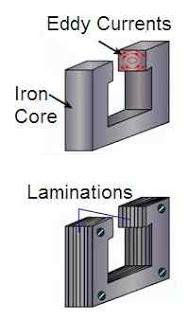# Eddy Current Loss Formula

Hi friends,

In this article, I am discussing the eddy current loss, eddy current loss formula, its causes and applications. So let us start with our topic.

## What is eddy current loss?

Whenever the magnetic flux linking to conducting material changes, voltages are induced in all possible paths and hence the induced currents are set up throughout the volume of the conducting material.

These currents are similar to the eddy-currents produced in water. Hence these current are called ‘eddy current’. In some cases, these currents may be so strong that the metal piece may become red-hot.

The electrical energy which is wasted in the form of heat due to eddy currents in the core material is called eddy current loss.

# Eddy Current Loss Formula | Equation

Eddy current loss, Pe = KeBmax2f2t2V watts

Where Ke = constant
Bmax = maximum flux density in Tesla
f  = frequency of magnetic reversal in Hz
t = thickness of laminations in mm
V = volume of core in m3

This eddy current loss formula or equation can be used in all electrical machines like transformers, AC and DC motors to calculate the eddy current loss.

The magnitude of these currents depends upon the resistance of the metal. If the resistance of the metal is large, then eddy currents are feeble.

These are produced in the interior of the iron cores of the rotating armatures of motors and dynamos, and also in the cores of transformers. Eddy current loss is undesirable in electrical machines as it produces heat and is responsible for the loss of energy thus producing additional temperature rise.

To minimize these currents, the cores of electrical machines are not taken as a single piece of soft-iron but are made of many thin laminas of soft-iron which are insulated from each other by varnish. This type of core is called the ‘laminated core’

In the laminated core, eddy currents are produced separately in all the laminas, instead of being produced in the entire volume of the solid core.

Thus, the length of the current path is appreciably increased, with a consequent increase in resistance. Hence the currents and their heating effect are minimized. The magnetization of the core, however, is not affected because the core is continuous in direction of the field.

## How can eddy current loss be minimized ?• At low frequencies, eddy current loss is reduced by making the core of a number of thin sheets, called laminations. They are lightly insulated from one another by special varnishes. It increases the resistance of the closed paths and reduces the eddy loss.
•

• This loss being proportional to the square of the frequency becomes excessively high at radio frequencies. It is not possible to make very thin laminations and hence granulated or powdered-iron cores are used. The iron dust is mixed with a binding material and compressed to form a required shape. Such cores are used at radio frequency operated devices.
•

• This loss can further be reduced by using the core material of a high resistivity. For this purpose, steel is alloyed with silicon up to 4%.

## Where is eddy current used ?

The eddy currents are usefully used in the following cases:

• Damping: The damping effect of eddy currents in used in some electrical measuring instruments. The coil of such instruments is wound on a light metal frame. When the coil and frame rotate in the field of the permanent magnet, the eddy currents set up in the frame provides damping torque in the instrument.
•

• Induction Furnace: In induction furnaces, the metal to be heated is placed in a rapidly changing magnetic field produced by a high-frequency alternating current. The eddy currents set up in the metal produce so much heat that the metal melts.
•

• Induction Motor: When a metallic cylinder is placed in a rotating magnetic field, eddy current set up in it. These currents, according to Lenz’s law, try to reduce the relative motion between the cylinder and the field. The cylinder, therefore, begins to rotate in the direction of the field. This is the principle of an induction motor.
•

• Electric Brakes: When a strong, stationary magnetic field is suddenly applied to a rotating drum, the eddy currents set up in the drum exert a torque which stops the motion of the drum. This principle is used in stopping electric trains.
•

• Besides these, eddy currents are employed in speedometers of automobiles and ‘deep heat treatment’ of the human body.

1.